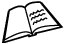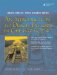# Simple Derivation• Chapter 2
• Chapter 5

Inheritance is a way of organizing classes that is supported by all object-oriented languages. It allows different classes to share code in many different ways.

To employ inheritance, we place the common features of similar classes together in a base class and then derive other classes from it. Each derived class inherits all the members of the base class and can override or extend each base class function as needed. Inheritance from a common base class significantly simplifes the derived classes and, with the use of certain design patterns, allows us to eliminate redundant code.## What s Wrong with repeated code?

Software that contains repeated pieces of identical or very similar code is error prone and difficult to maintain. If repeated code is allowed in a program, it can be difficult to keep track of all the repetitions.

Code often needs to be changed for one reason or another. If you need to change a piece of code that has been repeated several times, you must locate all of the repetitions and apply the change to each of them. Chances are good that at least one repetition will be missed or that the intended change will not be applied precisely to all repetitions.

Refactoring is a process of improving the design of software, without changing its underlying behavior. One step of refactoring involves replacing similar code with calls to library functions or base class methods.

We will demonstrate inheritance with a simple example. The base class Student is supposed to contain the attributes that are common to all students. We kept the list of attributes short for this example, but you can easily imagine other attributes that might be appropriate.

We derived two classes from Student that describe particular kinds of students. The first derived class, Undergrad, contains only those properties that are specific to undergraduate students. The second derived class, GradStudent, contains only those properties that are specific to graduate students. The UML diagram shown in Figure 6.1 describes these relationships.

Figure 6.1. UML diagram of inheritanceThe pound sign (#) that precedes Student::m_Year indicates that m_Year is a protected member of that class. Recall that protected members of a class are accessible to the member functions of derived classes. The open arrowhead (pointing at the base class) is used to indicate class inheritance. This arrow is also called generalization because it points from the more specific (derived) class to the more general (base) class. The derived classes are also called subclasses of the base class.

Example 6.1 shows the definitions of the three classes.

Example 6.1. src/derivation/qmono/student.h

 ```#ifndef STUDENT_H #define STUDENT_H #include class Student { public: Student(QString nm, long id, QString m_Major, int year = 1); ~Student() {} QString getClassName() const; <-- 1 QString toString() const; QString yearStr() const; private: QString m_Name; QString m_Major; long m_StudentId; protected: int m_Year; }; class Undergrad: public Student { public: Undergrad(QString name, long id, QString major, int year); QString getClassName() const; }; class GradStudent : public Student { public: enum Support { ta, ra, fellowship, other }; GradStudent(QString nm, long id, QString major, int yr, Support support); QString getClassName() const ; QString toString() const; protected: static QString supportStr(Support sup) ; private: Support m_Support; }; #endif // #ifndef STUDENT_H ```   (1)There are other ways of identifying the classname that are better than defining a getClassName() for each class. getClassName() is used here only to demonstrate how inheritance and polymorphism work.

The classHead of each derived class specifies the base class from which it is derived and the kind of derivation that is being used. In this case we are using public derivation.

 We discuss the three kinds of derivationpublic, protected, and privatein Section 23.4.

Notice that each of the three classes has a function named getClassName(), and two of them have a function named toString(). Even though Undergrad does not contain a toString() declaration in its definition, it inherits one from the base class.

The student functions are defined in Example 6.2.

Example 6.2. src/derivation/qmono/student.cpp

 ```[ . . . . ] #include #include "student.h" Student::Student(QString nm, long id, QString major, int year) : m_Name(nm), m_Major(major), m_StudentId(id), m_Year(year) {} QString Student::getClassName() const { return "Student"; } QString Student::toString() const { QString retval; QTextStream os(&retval); <-- 1 os << "[" << getClassName() << "]" << " name: " << m_Name << " Id: " << m_StudentId << " Year: " << yearStr() << " Major: " << m_Major ; return retval; } ```   (1)We write to the stream, and return the string it uses.

Undergrad is not very different from Student, except for one function: getClassName().

Example 6.3. src/derivation/qmono/student.cpp

 ```[ . . . . ] Undergrad::Undergrad(QString name, long id, QString major, int year) : Student(name, id, major, year) <-- 1 { } QString Undergrad::getClassName() const { return "Undergrad"; } ```   (1)The base class object is considered a subobject of the derived object. Class members and base classes both must be initialized and cleaned up, in an order determined by the order that they appear in the class definition.

Member Initialization for Base Classes

Because each Undergrad is a Student, whenever an Undergrad object is created, a Student object must also be created. In fact, one Student constructor is always called to initialize the Student part of any derived class. In the member initializers of a constructor, you can think of the base class name as an implicit member of the derived class.

• It gets initialized first, before the initialization of the derived class members.
• If you do not specify how the base class is initialized, the default constructor will be called.

GradStudent has all the features of Student plus some added attributes that need to be properly handled.

Example 6.4. src/derivation/qmono/student.cpp

 ```[ . . . . ] GradStudent:: GradStudent(QString nm, long id, QString major, int yr, Support support) :Student(nm, id, major, yr), m_Support(support) { } QString GradStudent::toString() const { QString result; QTextStream os(&result); os << Student::toString() <-- 1 << " [Support: " <-- 2 << supportStr(m_Support) << " ] "; return result; } ```   (1)base class version (2). . . plus items that are specific to GradStudent

Extending

Inside GradStudent::toString(), before the GradStudent attributes are printed, we explicitly call Student::toString(), which handles the base class attributes. In other words, GradStudent::toString() extends the functionality of Student::toString().

It is worth noting here that, since most of the data members of Student are private, we need a base class function (e.g., toString()) in order to access the base class data members. A GradStudent object cannot directly access the private members of Student even though it contains those members. This arrangement definitely takes some getting used to!

6.1.1. Inheritance Client Code Example

GradStudent is a Student, in the sense that a GradStudent object can be used wherever a Student object can be used. The client code shown in Example 6.5 creates some instances and performs operations on a GradStudent or an Undergrad instance directly and also indirectly, through pointers.

Example 6.5. src/derivation/qmono/student-test.cpp

 ```#include #include "student.h" static QTextStream cout(stdout, QIODevice::WriteOnly); void graduate(Student* student) { cout << " The following " << student->getClassName() << " has graduated " << student->toString() << " "; } int main() { Undergrad us("Frodo", 5562, "Ring Theory", 4); GradStudent gs("Bilbo", 3029, "History", 6, GradStudent::fellowship); cout << "Here is the data for the two students: "; cout << gs.toString() << endl; cout << us.toString() << endl; cout << " Here is what happens when they graduate: "; graduate(&us); graduate(&gs); return 0; } ```

To build this application we use qmake and make as follows:

```src/derivation/qmono> qmake -project
src/derivation/qmono> qmake
src/derivation/qmono> make
```

We then can run it like this:

```
```

[View full width]

src/derivation/qmono> ./qmono Here is the data for the two students: [Student] name: Bilbo Id: 3029 Year: gradualstudent Major: History [Support: fellowship] [Student] name: Frodo Id: 5562 Year: senior Major: Ring Theory Here is what happens when they graduate: The following Student has graduated [Student] name: Frodo Id: 5562 Year: senior Major: Ring Theory The following Student has graduated [Student] name: Bilbo Id: 3029 Year: gradual student Major: History src/derivation/qmono>

 It would be nice if we saw [GradStudent] here.

Calling student->toString() from the function graduate() invokes Student::toString() regardless of what kind of object student points to. If the object is, in fact, a GradStudent, then there should be a mention of the fellowship in the graduation message. In addition, we should be seeing "[GradStudent]" in the toString() messages, and we are not.

It would be more appropriate to use run-time binding for indirect function calls to determine which toString() is appropriate for each object.

Because of its C roots, C++ has a compiler that attempts to bind function invocations at compile time, for performance reasons. With inheritance and base class pointers, the compiler can have no way of knowing what type of object it is operating on. In the absence of run-time checking, an inappropriate function can be called. C++ requires the use of a special keyword to enable run-time binding on function calls via pointers and references. The keyword is virtual, and it enables polymorphism, which is explained in the next section.

### Derivation with PolymorphismAn Introduction to Design Patterns in C++ with Qt 4
ISBN: 0131879057
EAN: 2147483647
Year: 2004
Pages: 268